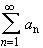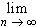#Interactive Real Analysis

Next | Previous | Glossary | Map

## 4.2. Convergence Tests

### Ratio Test

Consider the series. Then
• if lim sup | a n+1 / a n | < 1 then the series converges absolutely.
• if there exists an N such that | a n+1 / a n |1 for all n > N then the series diverges.
• if lim sup | a n+1 / a n | = 1, this test gives no information
Note that the second condition is true if lim | an+1 / an | exists and is strictly bigger than 1.
The ratio test is easier to use than the Root test . However, there are series for which the ratio tests gives no information, but the root test will. In that sense, the ratio test is weaker than the root test. In addition, the ratio test can be proved using the root test, but not visa versa.

Using the lim sup rather than the regular limit has the advantage that we don't have to worry about existence of the limit. However, if the regular limit exists, the lim sup yields the same number. Therefore, we loose nothing by looking at the limit superior.

 Example 4.2.16:Does Euler's seriesconverge ?Use the ratio test to test the seriesfor convergence. Compare with the same example using the root test.Are the following statements equivalent ? If not, which statement is stronger ? There exists an N such that | a n+1 / a n |1 for all n > N lim sup | a n+1 / a n |1Give an alternative proof of the ratio test using the root test (therefore showing that the root test is stronger than the ratio test).Use the ratio test and the divergence test to show that for any fixed number aan/n! = 0

### Proof:

The proof is very similar to the proof of the root test:

Assume that lim sup | a n+1 / a n | < 1: because of the properties of the lim sup, we know that there exists> 0 and N > 1 such that

| a n+1 / a n | < 1 -for n > N. Multiplying both sides by a n we obtain
| a n+1 | < (1-) | a n |
for n > N. Therefore, we also have
| a n+2 | < (1-) | a n+1 | < (1 -) 2 | a n |
for n > N. Repeating this procedure, we get, eventually, that
| a k | < (1 -) k-N | a N |
for k > N. But the terms on the right hand side form a convergent geometric series, indexed using the variable k, where N is some constant integer. Hence, by the comparison test the series with terms on the left-hand side will converge absolutely.

The proof for the second case if left as an exercise.Next | Previous | Glossary | Map